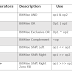Operators are special symbols that perform specific operations on one, two, or three operands, and then return a resultThere are different types of operators are available in java which are given below:
1. Arithmetic Operators.
2. Unary Operators.
3. Assignment Operators.
4. Relational Operators.
5. Logical Operators.
6. BitWise Operators.
7. Ternary Operators.
8. InstanceOf Operators.

## Bitwise Operators in Java with Example:

The Bitwise operators are used to perform a bit manipulation on numbers. This Operator can be used with any integral type(char, int, short, etc) but it cannot be applied to double and float. There are various Bitwise Operators used in Java:

## Types of Bitwise Operators in Java:

1. Bitwise AND (&) Operator: This operator returns 1 if both the operands are also 1 else it returns 0.

2. Bitwise OR (|) Operator: This operator returns 1 if either of the bits in the operand is 1, else it returns 0.

3. Bitwise Complement (~) Operator: This operator inverts all of the bits of its operands.

4. Bitwise Exclusive OR (^) Operator: This operator returns 1 if the corresponding bits are different, else it returns 0.

5. Bitwise Shift Left (<<) Operator: This operator shifts the bits of the number to the left and fills 0 in the void spaces that are left as a result.

6. Bitwise Shift Right (>>) Operator: This operator shifts the bits of the number to the right and fills 0 in the void spaces that are left as a result.

7. Shift Right Zero Fill (>>>), Operator: This operator shifts the bits of the number to the right and fills 0 in the void spaces that are left as a result. The leftmost bit is set to be 0.

### Example of Bitwise Operator in Java:

``````public class BitwiseOperator {
public static void main(String[] args)
{
//Initial values
int a = 6;
int b = 7;

// bitwise and
// 0110 & 0111=0110 = 6
System.out.println("a&b = " + (a & b));

// bitwise or
// 0110 | 0111=0111 = 7
System.out.println("a|b = " + (a | b));

// bitwise xor
// 0110 ^ 0111=0001 = 1
System.out.println("a^b = " + (a ^ b));

// bitwise and
// ~0110=1001
// will give 2's complement of 1001 = -7
System.out.println("~a = " + ~a);

// bitwise left shift
System.out.println("a << 2 = " +(a << 2));

// bitwise right shift
System.out.println("a >> 2 = " +(a >> 2));

// bitwise shift right zero fill
System.out.println("b >>> 2 = " +(b >>> 2));
}

}
```
```
You may also like these related posts:

BitWise Operators in Java with ExampleReviewed by Prashant Srivastava on December 15, 2019 Rating: 5